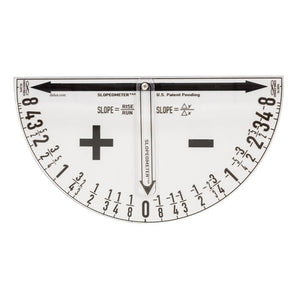# Didax Slopeometer, Magnetic, Slope Measurement (DD 211749)

Regular price \$29.99 \$0.00
The Slopeometer is a simple device to help students quickly and effectively learn and apply the mathematical concept of slope.

Made of sturdy, transparent plastic the Slopeometer securely attaches to any magnetic surface. Place the Slopeometer on the line being measured and the arrow moves to show the precise measurement of slope from 0 to infinity, positive and negative. From simple slope-intercept equations to the complex sketching of derivative functions in a calculus class, educators will find the Slopeometer an invaluable demonstration tool. Includes comprehensive activity guide.

Measures approximately 14” x 7 3/8”.